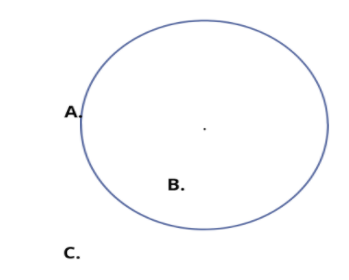Draw any circle and mark three points A,B and C such that(i) A is on the circle.(ii) B is in the interior of the circle.(iii) C is in the exterior of the circle.Verified
147.3k+ views
Hint: Draw a circle and plot the markings.

Complete step by step solution:(i) The point A is on the circle. it can be on any part of the circle margin.
The point is exactly at a radius distance from the centre
(ii) The B point is inside the circle. Any point inside the circle can be point B. The point is less than a
radius distance away from the centre.
(iii) Any point outside the circle can be point C. The point is outside the circle means that its distance
from the centre is more than the radius of the circle.Note: In a plane, the interior of a circle is the set of points whose distance from the centre is less than the radius. The exterior of a circle is the set of points in the plane whose distance from the centre is greater than the radius. Suppose the radius of the circle is 5 cm radius. Then when we put a point which is on the circle we mean to say that the point lies 5 cm from the centre. This point can be on any part of the circle at a distance of 5 cm. The same goes for a 6 cm radius or a 9 cm radius or any circle with any radius.